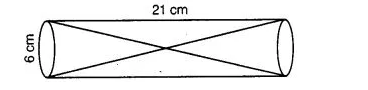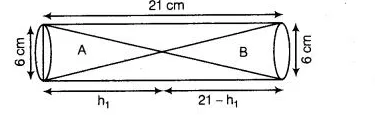# Two solid cones A and B are placed in a cylindrical

Question:

Two solid cones A and B are placed in a cylindrical tube as shown in the figure. The ratio of their capacities is 2 : 1. Find the heights and capacities of

cones. Also, find the volume of the remaining portion of the cylinder.Solution:

Let volume of cone A be 2 V and volume of cone B be V. Again, let height of the cone A = h1 cm, then height of cone B = (21 – h1) cmGiven, diameter of the cone $=6 \mathrm{~cm}$

$\therefore \quad$ Radius of the cone $=\frac{6}{2}=3 \mathrm{~cm}$

Now, volume of the cone, $A=2 V=\frac{1}{3} \pi r^{2} h=\frac{1}{3} \pi(3)^{2} h_{1}$

$\Rightarrow \quad V=\frac{1}{6} \pi 9 h_{1}=\frac{3}{2} h_{1} \pi$ $\ldots$ (i)

and volume of the cone, $B=V=\frac{1}{3} \pi(3)^{2}\left(21-h_{1}\right)=3 \pi\left(21-h_{1}\right)$ .....(ii)

From Eqs. (i) and (ii),

$\frac{3}{2} h_{1} \pi=3 \pi\left(21-h_{1}\right)$

$\Rightarrow \quad h_{1}=2\left(21-h_{1}\right)$

$\Rightarrow \quad 3 h_{1}=42$

$\Rightarrow \quad h_{1}=\frac{42}{3}=14 \mathrm{~cm}$

$\therefore \quad$ Height of cone, $B=21-h_{1}=21-14=7 \mathrm{~cm}$

Now, $\quad$ volume of the cone, $A=3 \times 14 \times \frac{22}{7}=132 \mathrm{~cm}^{3}$ [using Eq. (i)]

and $\quad$ volume of the cone, $B=\frac{1}{3} \times \frac{22}{7} \times 9 \times 7=66 \mathrm{~cm}^{3}$ [using Eq. (ii)]

Now, $\quad$ volume of the cylinder $=\pi r^{2} h=\frac{22}{7}(3)^{2} \times 21=594 \mathrm{~cm}^{3}$

$\therefore$ Required volume of the remaining portion $=$ Volume of the cylinder

- (Volume of cone $A+$ Volume of cone $B$ )

$=594-(132+66)$

$=396 \mathrm{~cm}^{3}$